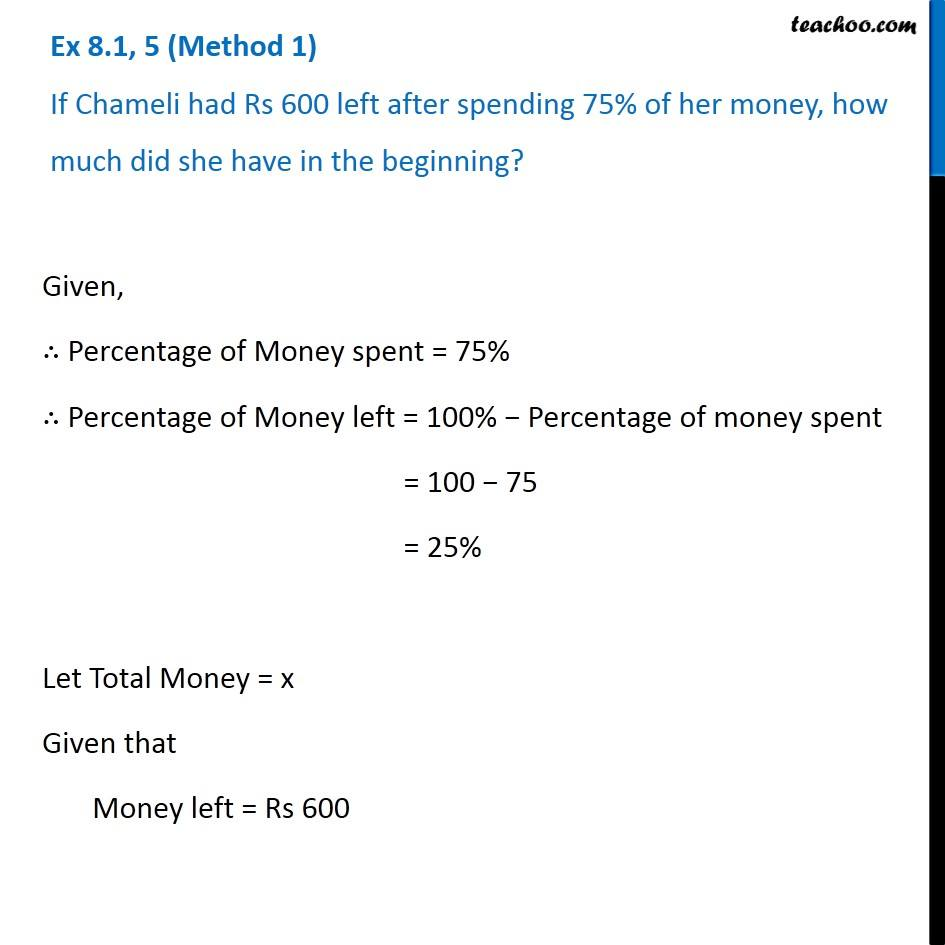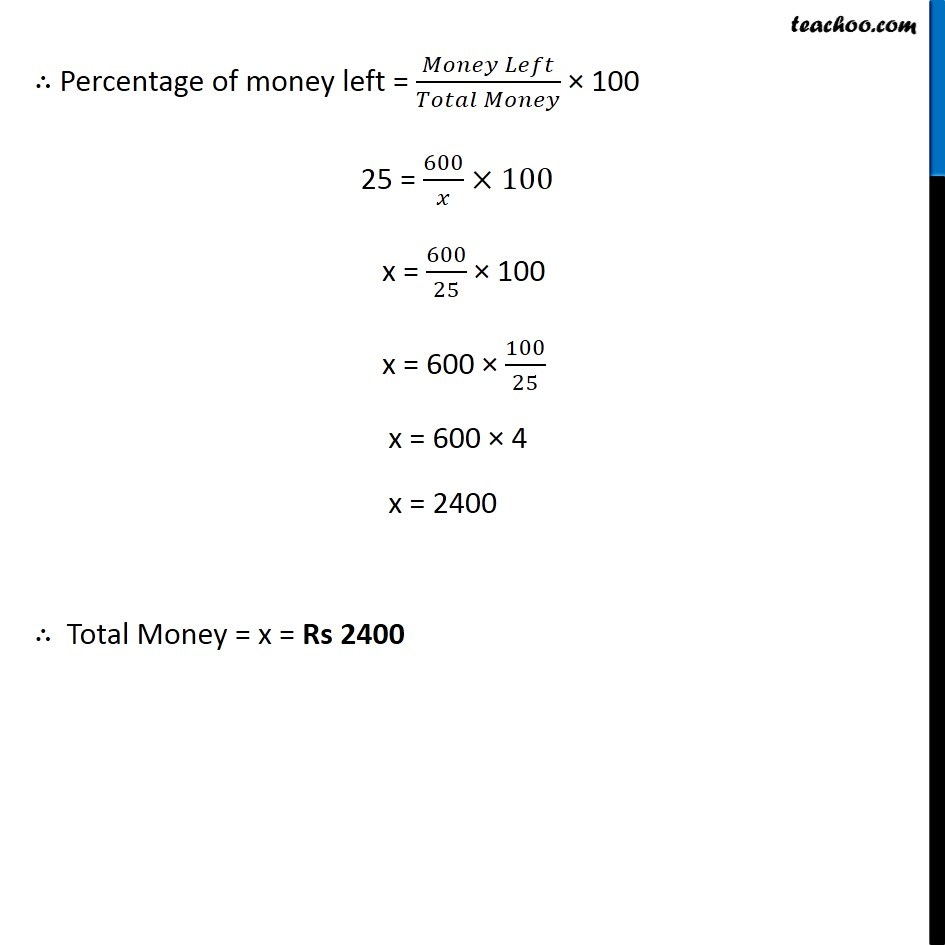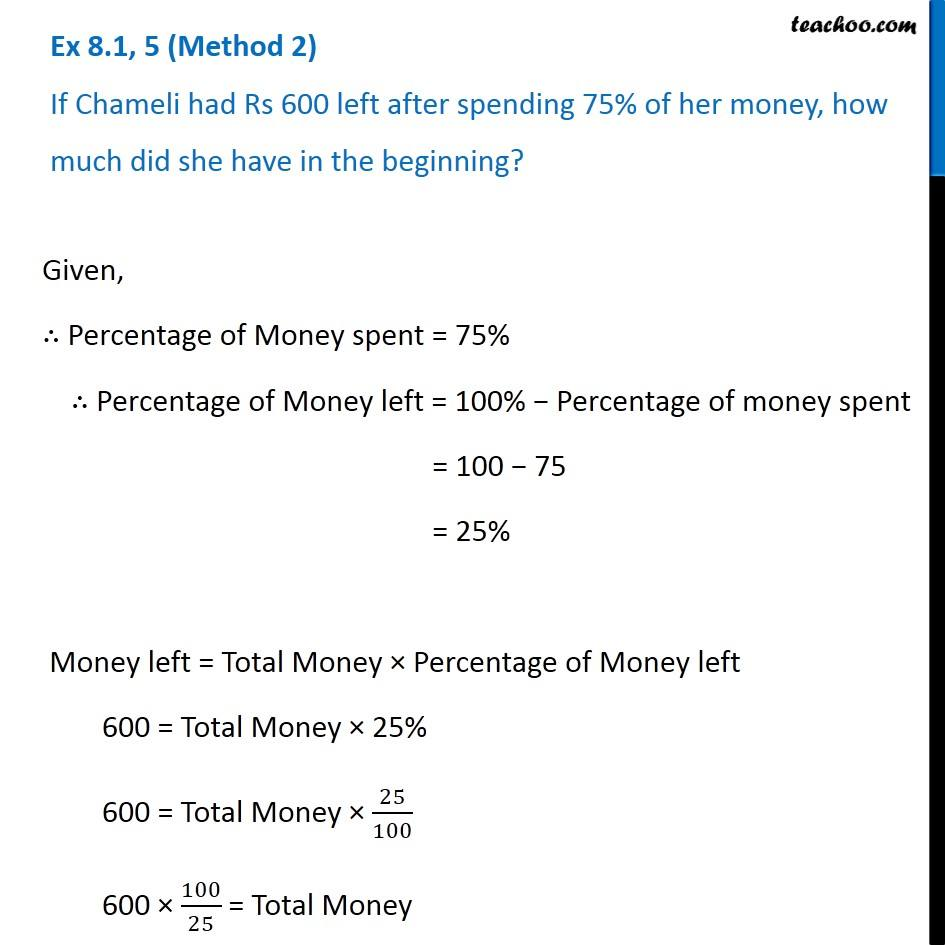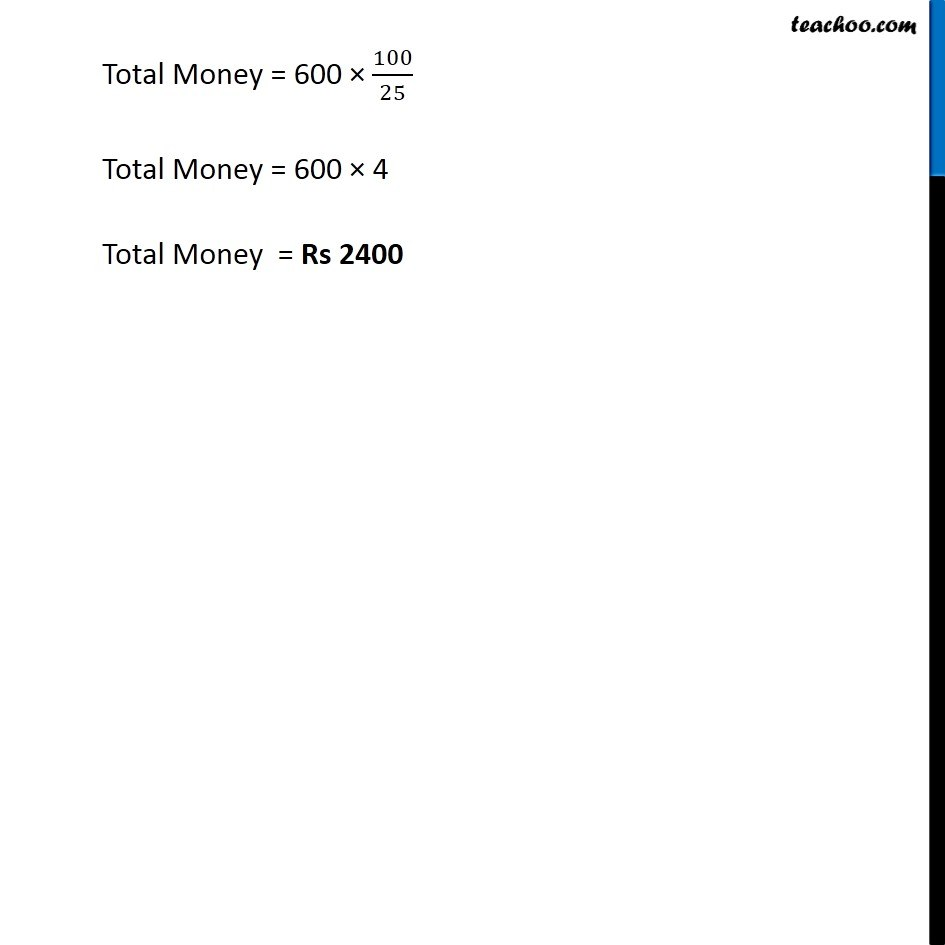Ex 7.1

Chapter 7 Class 8 Comparing Quantities
Serial order wiseLearn in your speed, with individual attention - Teachoo Maths 1-on-1 Class

### Transcript

Ex 7.1, 5 (Method 1) If Chameli had Rs 600 left after spending 75% of her money, how much did she have in the beginning? Given, ∴ Percentage of Money spent = 75% ∴ Percentage of Money left = 100% − Percentage of money spent = 100 − 75 = 25% Let Total Money = x Given that Money left = Rs 600 ∴ Percentage of money left = (𝑀𝑜𝑛𝑒𝑦 𝐿𝑒𝑓𝑡)/(𝑇𝑜𝑡𝑎𝑙 𝑀𝑜𝑛𝑒𝑦) × 100 25 = 600/𝑥×100 x = 600/25 × 100 x = 600 × 100/25 x = 600 × 4 x = 2400 ∴ Total Money = x = Rs 2400 Ex 7.1, 5 (Method 2) If Chameli had Rs 600 left after spending 75% of her money, how much did she have in the beginning? Given, ∴ Percentage of Money spent = 75% ∴ Percentage of Money left = 100% − Percentage of money spent = 100 − 75 = 25% Money left = Total Money × Percentage of Money left 600 = Total Money × 25% 600 = Total Money × 25/100 600 × 100/25 = Total Money Total Money = 600 × 100/25 Total Money = 600 × 4 Total Money = Rs 2400